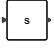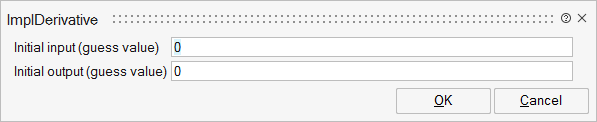# ImplDerivative

This block outputs the derivative of its input signal. The solver uses a multi-order, multi-step BDF, Backward Differentiation Formula to estimate the derivative. The accuracy of this method is higher than the "du/dt" block which uses an order 1 method. The accuracy can be improved by reducing the solver error tolerances.Dynamical

## Description

The ImplDerivative block outputs the derivative of its input signal. The solver uses a multi-order, multi-step BDF (backward differentiation formula) method to estimate the derivative. The accuracy of this method is higher than the "du/dt" block which uses an order 1 method. The accuracy can be improved by reducing the solver error tolerances.

## ParametersNameLabelDescriptionData TypeValid Values

x0

Initial input (guess value)

The initial value of the state is used as an initial guess to help the solver converge toward the solution. This is especially useful at the start of the simulation.

Matrix

xd0

Initial output (guess value)

The initial value of the state derivative is used as an initial guess to help the solver converge. The very first output of the block is the initial derivative vector.

Matrix

## Ports

NameTypeDescriptionIO TypeNumber

Port 1

explicit

output

1

Port 2

explicit

input

1

NameValueDescription

always active

yes

direct-feedthrough

no

zero-crossing

no

mode

no

continuous-time state

yes

discrete-time state

no